## 2. 5. 5 Multi-Argument Functions

Hitherto, the argument which is passed to a function was treated in a straight forward manner. Functions pass the argument given to the compound functions, these functions are evaluated and afterwards, an operation is performed using the result of the single evaluation of the compound functions, for instance an addition. For the summation an equivalent problem occurs. As a first example, which shall introduce the use of unnamed functions, a binary function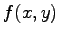is given. A compound function shall be written which yields the following result: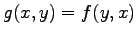.

Due to the possibility of altering the argument of the quantity functions, functions are not restricted to the evaluation of one single argument. As a consequence it can also be sensible to use a binary second order function. Here, the unnamed functions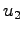andrepresent the following dependences: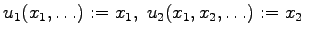(2.41)

This can be generalized in the following manner: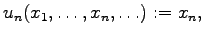(2.42)

In order to specify the required commutation function, one can write the following functional expression: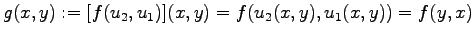(2.43)

In order not to complicate the already introduced one-argument formalisms, it is generally assumed that all arguments are passed to the respective function in the correct order. Quantities can therefore be evaluated with respect to one of the passed arguments. In this case, the respective second order quantity function (for the sake of explicitness, the second order function is written as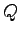) can be evaluated.

If the second-order quantity functionis evaluated with respect to the first argument it can be written shortly as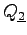or only as. An evaluation with the second-order function is written by the abbreviation. Using this notation, the following expressions hold true: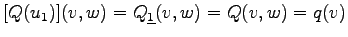(2.44)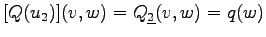(2.45)

If quantities functions are evaluated within the scope of a binary function, expressions can be formed in the following manner: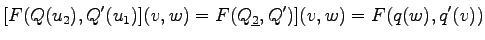(2.46)

If higher order functions are used with unnamed functions as arguments in the natural order such as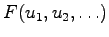, the notation can be replaced by writing bullets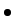instead of the unnamed functions. This abbreviation leads to the following formalisms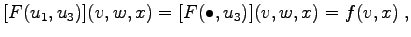(2.47)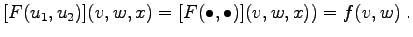(2.48)

It can be seen that the consequent passing of arguments to a function is used as default, whereas deviations from this standard have to be specified explicitly. The following binary function shows the determination of the number of common vertices of two simplicial cells, for instance tetrahedra. This can be necessary, whether it has to be determined, if two cells have a common edge (two common vertices), a common facet (three common vertices), or they are identical (all four vertices are identical). As an auxiliary function, the Kronecker symbol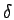function is used. If two elements are identical, this function returns unity, otherwise zero. The respective second order function is called.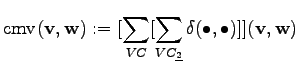(2.49)

As for all other elements of this calculus, also the summations are second order functions. Hitherto, it has been implicitly assumed, that the base element of the summation is the argument passed to the sum function. As two arguments are available, it has to be specified which argument is used. In order to treat sums as any other second order function, a function is passed to the summation function, which determines the base element of the summation from the arguments passed.

Michael 2008-01-16